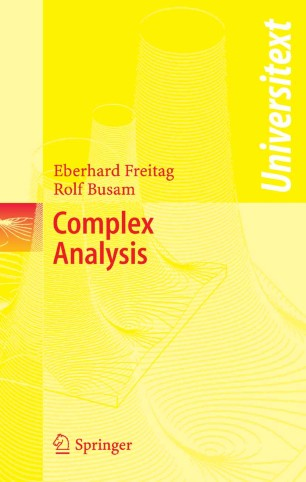# Complex Analysis

## Benefits

• All needed notions are developed within the book: with the exception of fundamentals which are presented in introductory lectures, no other knowledge is assumed

• Provides a more in-depth introduction to the subject than other existing books in this area

• Over 400 exercises including hints for solutions are includedTextbook

Part of the Universitext book series (UTX)

1. Front Matter
Pages I-X
2. Pages 1-7
3. Pages 71-104
4. Pages 195-255
5. Pages 257-325
6. Pages 327-390
7. Pages 391-458
8. Pages 459-521
9. Back Matter
Pages 523-552

### Introduction

The guiding principle of this presentation of ``Classical Complex Analysis'' is to proceed as quickly as possible to the central results while using a small number of notions and concepts from other fields. Thus the prerequisites for understanding this book are minimal; only elementary facts of calculus and algebra are required.

The first four chapters cover the essential core of complex analysis:

-  differentiation in  C  (including elementary facts about conformal mappings)
-  integration in C   (including complex line integrals, Cauchy's Integral Theorem, and the Integral Formulas)
-  sequences and series of analytic functions,   (isolated) singularities, Laurent series, calculus of residues
-  construction of analytic functions:  the gamma function, Weierstrass' Factorization Theorem, Mittag-Leffler Partial Fraction Decomposition, and -as a particular highlight- the Riemann Mapping Theorem, which characterizes the simply connected domains in C.

Further topics included are:

- the theory of elliptic functions based on the model of K. Weierstrass (with an excursions to older approaches due to N.H. Abel and C.G.J. Jacobi using theta series)
-  an introduction to the theory of elliptic modular functions and elliptic modular forms
-  the use of complex analysis to obtain number theoretical results
-  a proof of the Prime Number Theorem with a weak form of the error term.

The book is especially suited for graduated students in mathematics and advanced undergraduated students in mathematics and other sciences.

Motivating introductions, more than four hundred exercises of all levels of difficulty with hints or solutions, historical annotations, and over 120 figures make the overall presentation very attractive. The structure of the text, including abstracts beginning each chapter and highlighting of the main results, makes this book very appropriate for self-guided study and an indispensable aid in preparing for tests.

This English edition is based on the fourth forthcoming German edition.

### Keywords

Complex analysis Elliptic Functions Elliptic Modular Forms Modular form Residue theorem calculus minimum

#### Authors and affiliations

1. 1.Faculty of Mathematics, Institute of MathematicsUniversity of HeidelbergHeidelbergGermany

### Bibliographic information

• Book Title Complex Analysis
• Authors Eberhard Freitag
Rolf Busam
• Series Title Universitext
• DOI https://doi.org/10.1007/3-540-30823-7
• Copyright Information Springer-Verlag Berlin Heidelberg 2005
• Publisher Name Springer, Berlin, Heidelberg
• eBook Packages Mathematics and Statistics Mathematics and Statistics (R0)
• Softcover ISBN 978-3-540-25724-0
• eBook ISBN 978-3-540-30823-2
• Edition Number 1
• Number of Pages X, 552
• Number of Illustrations 110 b/w illustrations, 2 illustrations in colour
• Topics
• Buy this book on publisher's site

## Reviews

From the reviews:

"The guiding principle of the presentation of classical complex analysis is to proceed as quickly as possible to the central results while using a small number of notions and concepts from other fields. Thus the prerequisites for understanding this book are minimal; only elementary facts of calculus and algebra are required. … Motivating introductions, more than four hundred exercises of all levels of difficulty with hints or solutions, historical annotations, and over 120 figures make the overall presentation very attractive." (L’Enseignement Mathematique, Vol. 52 (2), 2006)

"The first four chapters cover the essential core of complex analysis … . The second part of the book is devoted to an extensive representation of the theory of elliptic functions … . Interesting introductions, over four hundred exercises with hints or solutions, historical remarks, and over 120 figures make this book very appropriate and attractive for students at all levels." (F. Haslinger, Monatshefte für Mathematik, Vol. 149 (3), 2006)

"It is, in fact, a massive introduction to complex analysis, covering a very wide range of topics. … This is the material that I like to cover in an undergraduate course. … Theorems and proofs are clearly delimited, which many students find helpful. … There are problems at the end of each section, and sketches of solutions are given … . Overall, this is quite an attractive book." (Fernando Q. Gouvêa, MathDL, February, 2006)

“This book gives an extensive presentation of the theory of classical complex analysis. … The book is richly illustrated with a lot of exercises (more than 400), including solutions … with a wide range of levels of difficulties. The historical annotations, the large amount of figures and the helpful abstracts at the beginning of each chapter make this book very attractive. … recommended to graduated (or advanced undergraduated) students who wish to proceed quickly to the main results of complex analysis.” (Samuel Nicolay, Bulletin of the Belgian Mathematical Society, Vol. 15 (1), 2008)